## Page Fault in OS-

Before you go through this article, make sure that you have gone through the previous article on Page Fault in OS.

We have discussed-

• When a page fault occurs, the required page has to be brought from the secondary memory.
• If all the frames of main memory are already occupied, then a page has to be replaced.
• Page Replacement Algorithms help to decide which page should be replaced.

## Effect of Increasing Number of Frames-

• The number of page faults should either decrease or remain constant on increasing the number of frames in main memory.
• But sometimes the unusual behavior is observed.
• Sometimes, on increasing the number of frames in main memory, the number of page faults also increase.

Belady’s Anomaly is the phenomenon of increasing the number of page faults on increasing the number of frames in main memory.

Following page replacement algorithms suffer from Belady’s Anomaly-

• FIFO Page Replacement Algorithm
• Random Page Replacement Algorithm
• Second Chance Algorithm

## NOTE-

In the above discussion,

• “Algorithms suffer from Belady’s Anomaly” does not mean that always the number of page faults will increase on increasing the number of frames in main memory.
• This unusual behavior is observed only sometimes.

• An algorithm suffers from Belady’s Anomaly if and only if it does not follow stack property.
• Algorithms that follow stack property are called as stack based algorithms.
• Stack based algorithms do not suffer from Belady’s Anomaly.
• This is because these algorithms assign priority to a page for replacement that is independent of the number of frames in the main memory.

## Examples-

Following page replacement algorithms are stack based algorithms-

1. LRU Page Replacement Algorithm
2. Optimal Page Replacement Algorithm

Hence, they do not suffer from Belady’s Anomaly.

## Stack Property-

Consider-

• Initially, we had ‘m’ number of frames in the main memory.
• Now, the number of frames in the main memory is increased to ‘m+1’.

According to stack property-

• At each stage, the set of pages that were present in the main memory when number of frames is ‘m’ will be compulsorily present in the corresponding stages in main memory when the number of frames is increased to ‘m+1’.

The following illustrations explain it clearly.

## Illustration-01: For Optimal Page Replacement Algorithm-

Consider the reference string is-

0, 1, 2, 3, 0, 1, 4, 0, 1, 2, 3, 4

Now, observe the following two cases-

### Case-01: When frame size = 3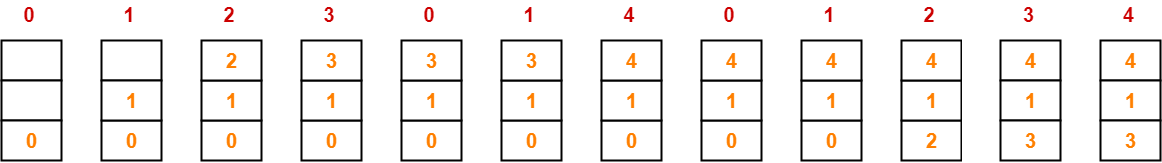Number of page faults = 7

### Case-02: When frame size = 4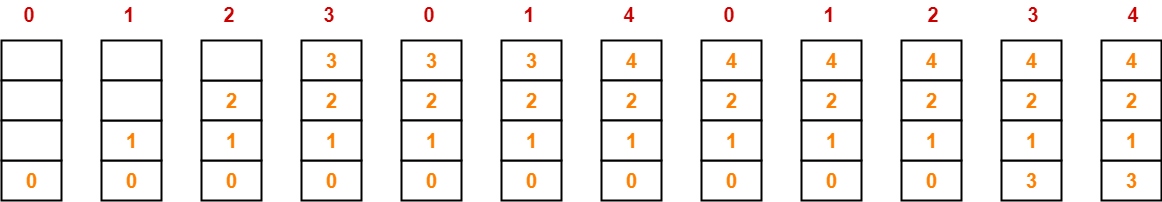Number of page faults = 6

From here, we can observe-

• At all the stages in case-02, main memory compulsorily contains the set of pages that are present in the corresponding stages in case-01.
• Thus, optimal page replacement algorithm follows the stack property.
• Hence, it does not suffer from Belady’s Anomaly.
• As a proof, number of page faults decrease when the number of frames is increased from 3 to 4.

## Illustration-02: For LRU Page Replacement Algorithm-

Consider the reference string is-

0, 1, 2, 3, 0, 1, 4, 0, 1, 2, 3, 4

Now, observe the following two cases-

### Case-01: When frame size = 3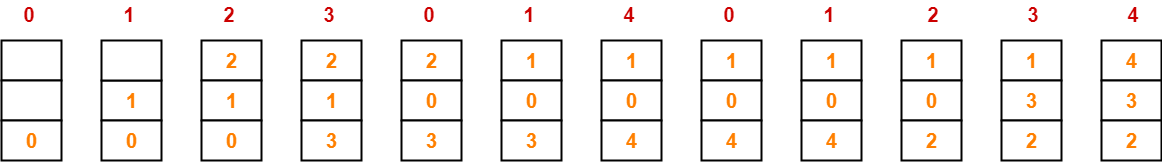Number of page faults = 10

### Case-02: When frame size = 4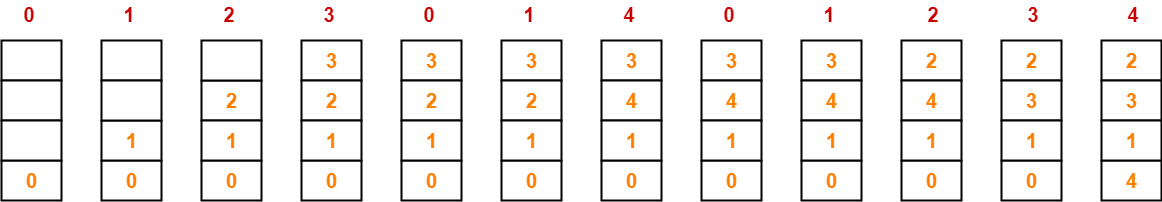Number of page faults = 8

From here, we can observe-

• At all the stages in case-02, main memory compulsorily contains the set of pages that are present in the corresponding stages in case-01.
• Thus, LRU page replacement algorithm follows the stack property.
• Hence, it does not suffer from Belady’s Anomaly.
• As a proof, number of page faults decrease when the number of frames is increased from 3 to 4.

## Illustration-03: For FIFO Page Replacement Algorithm-

Consider the reference string is-

0, 1, 2, 3, 0, 1, 4, 0, 1, 2, 3, 4

Now, observe the following two cases-

### Case-01: When frame size = 3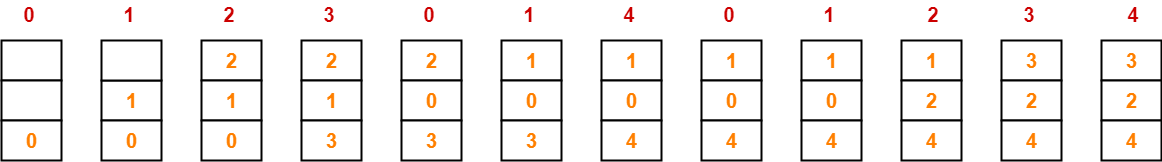Number of page faults = 9

### Case-02: When frame size = 4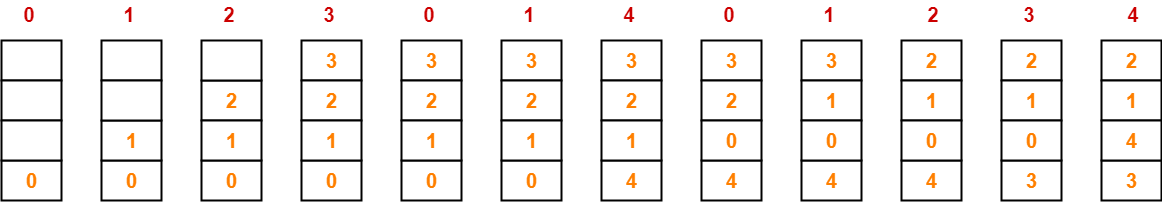Number of page faults = 10

From here, we can observe-

• At stage-07 and stage-08 in case-02, main memory does not contain the set of pages that are present in the corresponding stages in case-01.
• Thus, FIFO page replacement algorithm does not follow the stack property.
• Hence, it suffers from Belady’s Anomaly.
• As a proof, number of page faults increase when the number of frames is increased from 3 to 4.

## NOTE-

In the above illustration,

• FIFO Page Replacement Algorithm suffers from Belady’s Anomaly.
• It would be wrong to say that “FIFO Page Replacement Algorithm always suffer from Belady’s Anomaly”.

Watch this Video Lecture

Next Article- Page Replacement Algorithms Important Results

Get more notes and other study material of Operating System.

Watch video lectures by visiting our YouTube channel LearnVidFun.

## Page Fault in OS-

Before you go through this article, make sure that you have gone through the previous article on Page Fault in OS.

We have discussed-

• A page fault occurs when a page referenced by the CPU is not found in the main memory.
• The required page has to be brought from the secondary memory into the main memory.
• A page has to be replaced if all the frames of main memory are already occupied.

## Page Replacement-

 Page replacement is a process of swapping out an existing page from the frame of a main memory and replacing it with the required page.

Page replacement is required when-

• All the frames of main memory are already occupied.
• Thus, a page has to be replaced to create a room for the required page.

## Page Replacement Algorithms-

Page replacement algorithms help to decide which page must be swapped out from the main memory to create a room for the incoming page.

Various page replacement algorithms are-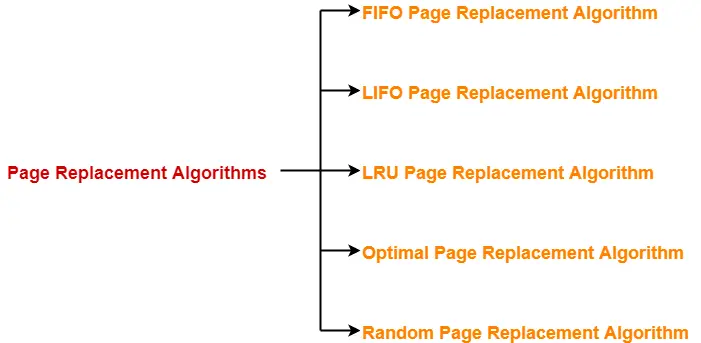1. FIFO Page Replacement Algorithm
2. LIFO Page Replacement Algorithm
3. LRU Page Replacement Algorithm
4. Optimal Page Replacement Algorithm
5. Random Page Replacement Algorithm

 A good page replacement algorithm is one that minimizes the number of page faults.

## FIFO Page Replacement Algorithm-

• As the name suggests, this algorithm works on the principle of “First in First out“.
• It replaces the oldest page that has been present in the main memory for the longest time.
• It is implemented by keeping track of all the pages in a queue.

## LIFO Page Replacement Algorithm-

• As the name suggests, this algorithm works on the principle of “Last in First out“.
• It replaces the newest page that arrived at last in the main memory.
• It is implemented by keeping track of all the pages in a stack.

### NOTE

Only frame is used for page replacement during entire procedure after all the frames get occupied.

## LRU Page Replacement Algorithm-

• As the name suggests, this algorithm works on the principle of “Least Recently Used“.
• It replaces the page that has not been referred by the CPU for the longest time.

## Optimal Page Replacement Algorithm-

• This algorithm replaces the page that will not be referred by the CPU in future for the longest time.
• It is practically impossible to implement this algorithm.
• This is because the pages that will not be used in future for the longest time can not be predicted.
• However, it is the best known algorithm and gives the least number of page faults.
• Hence, it is used as a performance measure criterion for other algorithms.

## Random Page Replacement Algorithm-

• As the name suggests, this algorithm randomly replaces any page.
• So, this algorithm may behave like any other algorithm like FIFO, LIFO, LRU, Optimal etc.

## Problem-01:

A system uses 3 page frames for storing process pages in main memory. It uses the First in First out (FIFO) page replacement policy. Assume that all the page frames are initially empty. What is the total number of page faults  that will occur while processing the page reference string given below-

4 , 7, 6, 1, 7, 6, 1, 2, 7, 2

Also calculate the hit ratio and miss ratio.

## Solution-

Total number of references = 10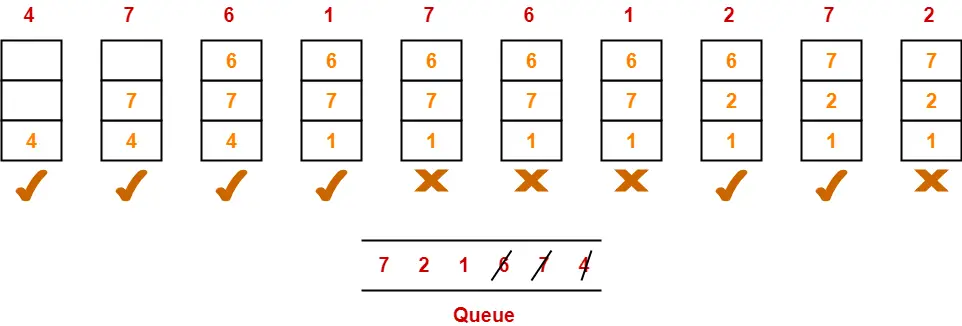From here,

Total number of page faults occurred = 6

### Calculating Hit ratio-

Total number of page hits

= Total number of references – Total number of page misses or page faults

= 10 – 6

= 4

Thus, Hit ratio

= Total number of page hits / Total number of references

= 4 / 10

= 0.4 or 40%

### Calculating Miss ratio-

Total number of page misses or page faults = 6

Thus, Miss ratio

= Total number of page misses / Total number of references

= 6 / 10

= 0.6 or 60%

Alternatively,

Miss ratio

= 1 – Hit ratio

= 1 – 0.4

= 0.6 or 60%

## Problem-02:

A system uses 3 page frames for storing process pages in main memory. It uses the Least Recently Used (LRU) page replacement policy. Assume that all the page frames are initially empty. What is the total number of page faults  that will occur while processing the page reference string given below-

4 , 7, 6, 1, 7, 6, 1, 2, 7, 2

Also calculate the hit ratio and miss ratio.

## Solution-

Total number of references = 10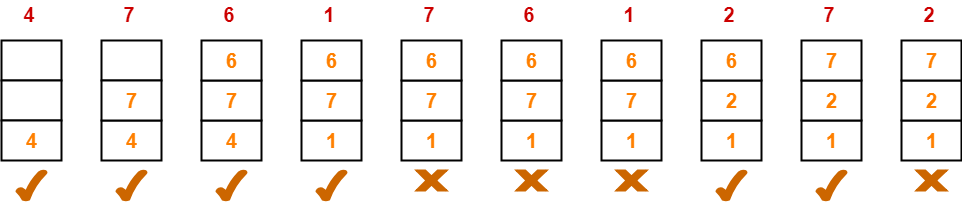From here,

Total number of page faults occurred = 6

In the similar manner as above-

• Hit ratio = 0.4 or 40%
• Miss ratio = 0.6 or 60%

## Problem-03:

A system uses 3 page frames for storing process pages in main memory. It uses the Optimal page replacement policy. Assume that all the page frames are initially empty. What is the total number of page faults  that will occur while processing the page reference string given below-

4 , 7, 6, 1, 7, 6, 1, 2, 7, 2

Also calculate the hit ratio and miss ratio.

## Solution-

Total number of references = 10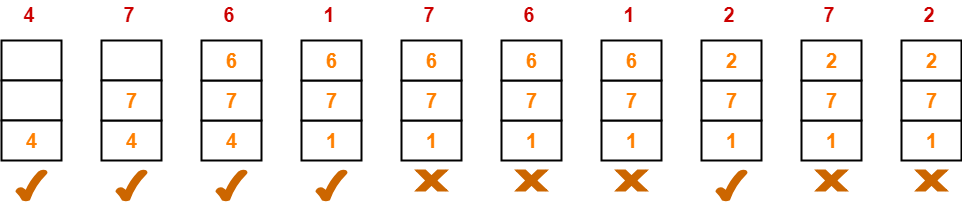From here,

Total number of page faults occurred = 5

In the similar manner as above-

• Hit ratio = 0.5 or 50%
• Miss ratio = 0.5 or 50%

To gain better understanding about Page Replacement Algorithms,

Watch this Video Lecture

Next Article- Practice Problems On Page Fault

Get more notes and other study material of Operating System.

Watch video lectures by visiting our YouTube channel LearnVidFun.

## Page Fault in OS-

Before you go through this article, make sure that you have gone through the previous article on Page Fault in OS.

We have discussed-

• A page fault occurs when the referenced page is not found in the main memory.
• Page fault handling routine is executed on the occurrence of page fault.
• The time taken to service the page fault is called as page fault service time.

## Effective Access time-

In a multilevel paging scheme using TLB without any possibility of page fault, effective access time is given by-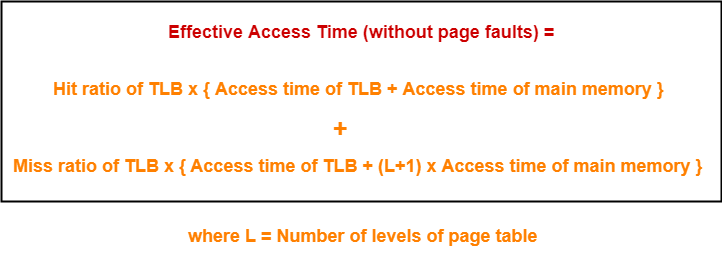In a multilevel paging scheme using TLB with a possibility of page fault, effective access time is given by-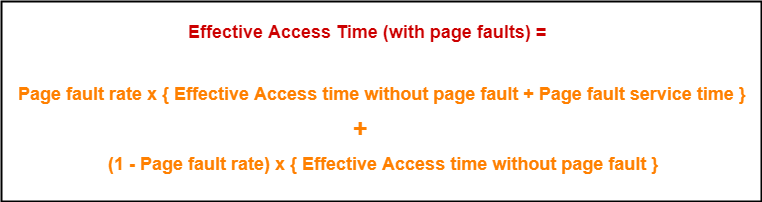## Problem-01:

Let the page fault service time be 10 ms in a computer with average memory access time being 20 ns. If one page fault is generated for every 106 memory accesses, what is the effective access time for the memory?

1. 21 ns
2. 30 ns
3. 23 ns
4. 35 ns

## Solution-

Given-

• Page fault service time = 10 ms
• Average memory access time = 20 ns
• One page fault occurs for every 106 memory accesses

### Page Fault Rate-

It is given that one page fault occurs for every 106 memory accesses.

Thus,

Page fault rate

= 1 / 106

= 10-6

### Effective Access Time With Page Fault-

It is given that effective memory access time without page fault = 20 ns.

Now, substituting values in the above formula, we get-

Effective access time with page fault

= 10-6 x { 20 ns + 10 ms } + ( 1 – 10-6 ) x { 20 ns }

= 10-6 x 10 ms + 20 ns

= 10-5 ms + 20 ns

= 10 ns + 20 ns

= 30 ns

Thus, Option (B) is correct.

## Problem-02:

Suppose the time to service a page fault is on the average 10 milliseconds, while a memory access takes 1 microsecond. Then, a 99.99% hit ratio results in average memory access time of-

1. 1.9999 milliseconds
2. 1 millisecond
3. 9.999 microseconds
4. 1.9999 microseconds
5. None of these

## Solution-

Given-

• Page fault service time = 10 msec
• Average memory access time = 1 μsec
• Hit ratio = 99.99% = 0.9999

Page fault rate

= 1 – Hit ratio

= 1 – 0.9999

= 0.0001

### Effective Access Time With Page Fault-

It is given that effective memory access time without page fault = 1 μsec.

Substituting values in the above formula, we get-

Effective access time with page fault

= 0.0001 x { 1 μsec + 10 msec } + 0.99999 x 1 μsec

= 0.0001 μsec + 0.001 msec + 0.9999 μsec

= 1 μsec + 0.001 msec

= 1 μsec + 1 μsec

= 2 μsec or 0.002 msec

Thus, Option (E) is correct.

## Problem-03:

If an instruction takes i microseconds and a page fault takes an additional j microseconds, the effective instruction time if on the average a page fault occurs every k instruction is-

1. i + j / k
2. i + j x k
3. (i + j) / k
4. (i + j) x k

## Solution-

Given-

• Page fault service time = j μsec
• Average memory access time = i μsec
• One page fault occurs every k instruction

### Page Fault Rate-

It is given that one page fault occurs every k instruction.

Thus,

Page fault rate

= 1 / k

### Effective Access Time With Page Fault-

It is given that effective memory access time without page fault = i μsec

Now, substituting values in the above formula, we get-

Effective access time with page fault

= (1 / k) x { i μsec + j μsec } + ( 1 – 1 / k) x { i μsec }

= j / k μsec + i μsec

= i + j / k μsec

Thus, Option (A) is correct.

## Problem-04:

Consider a system with a two-level paging scheme in which a regular memory access takes 150 nanoseconds and servicing a page fault takes 8 milliseconds. An average instruction takes 100 nanoseconds of CPU time and two memory accesses. The TLB hit ratio is 90% and the page fault rate is one in every 10,000 instructions. What is the effective average instruction execution time?

1. 645 nanoseconds
2. 1050 nanoseconds
3. 1215 nanoseconds
4. 1230 nanoseconds
5. None of these

## Solution-

Given-

• Number of levels of page table = 2
• Main memory access time = 150 ns
• Page fault service time = 8 msec
• Average instruction takes 100 ns of CPU time and 2 memory accesses
• TLB Hit ratio = 90% = 0.9
• Page fault rate = 1 / 104 = 10-4

Assume TLB access time = 0 since it is not given in the question.

Also, TLB access time is much less as compared to the memory access time.

### Effective Access Time Without Page Fault-

Substituting values in the above formula, we get-

Effective memory access time without page fault

= 0.9 x { 0 + 150 ns } + 0.1 x { 0 + (2+1) x 150 ns }

= { 0.9 x 150 ns } + { 0.1 x 450 ns }

= 135 ns + 45 ns

= 180 ns

### Effective Access Time With Page Fault-

Substituting values in the above formula, we get-

Effective access time with page fault

= 10-4 x { 180 ns + 8 msec } + (1 – 10-4) x 180 ns

= 8 x 10-4 msec + 180 ns

= 8 x 10-7 sec + 180 ns

= 800 ns + 180 ns

= 980 ns

### Effective Average Instruction Execution Time-

Effective Average Instruction Execution Time

= 100 ns + 2 x Effective memory access time with page fault

= 100 ns + 2 x 980 ns

= 100 ns + 1960 ns

= 2060 ns

Thus, Option (E) is correct.

## Problem-05:

A demand paging system takes 100 time units to service a page fault and 300 time units to replace a dirty page. Memory access time is 1 time unit. The probability of a page fault is p. In case of a page fault, the probability of page being dirty is also p. It is observed that the average access time is 3 time units. Then the value of p is-

1. 0.194
2. 0.233
3. 0.514
4. 0.981
5. None of these

## Solution-

Given-

• Page fault service time = 100 time units
• Time taken to replace dirty page = 300 time units
• Average memory access time = 1 time unit
• Page fault rate = p
• Probability of page being dirty = p
• Effective access time = 3 time units

Now, According to question-

3 time units = p x { 1 time unit + p x { 300 time units } + (1 – p) x { 100 time units } } + (1 – p) x { 1 time unit }

3 = p x { 1 + 300p + 100 – 100p } + (1 – p)

3 = p x { 101 + 200p } + (1 – p)

3 = 101p + 200p2 + 1 – p

3 = 100p + 200p2 + 1

200p2 + 100p – 2 = 0

On solving this quadratic equation, we get p = 0.019258

Thus, Option (E) is correct.

Get more notes and other study material of Operating System.

Watch video lectures by visiting our YouTube channel LearnVidFun.

## Paging in OS-

Before you go through this article, make sure that you have gone through the previous article on Paging in OS.

We have discussed-

• Paging is a non-contiguous memory allocation technique.
• In a paging scheme, a process is divided into several pages.
• The pages are then stored in different frames of the main memory.

## Page Fault-

• When a page referenced by the CPU is not found in the main memory, it is called as a page fault.
• When a page fault occurs, the required page has to be fetched from the secondary memory into the main memory.

In a paging scheme using TLB with possibility of page fault,

The logical address generated by the CPU is translated into the physical address using the following steps-

## Step-01:

CPU generates a logical address consisting of two parts-

1. Page Number
2. Page Offset

## Step-02:

• TLB is checked to see if it contains an entry for the referenced page number.
• The referenced page number is compared with the TLB entries all at once.

Now, two cases are possible-

### Case-01: If there is a TLB hit-

• If TLB contains an entry for the referenced page number, a TLB hit occurs.
• In this case, TLB entry is used to get the frame number for the referenced page number.

### Case-02: If there is a TLB miss-

• If TLB does not contain an entry for the referenced page number, a TLB miss occurs.
• In this case, page table is used to get the frame number for the referenced page number.
• The valid / invalid bit of the page table entry indicates whether the referenced page is present in the main memory or not.

Now, two cases are possible-

### Case-01: If Valid / Invalid Bit is Set to 1-

• If valid / invalid bit is set to 1, it indicates that the page is present in the main memory.
• Then, page table is used to get the frame number for the referenced page number.
• Then, TLB is updated with the page number and its frame number for future references.

### Case-02: If Valid / Invalid Bit is Set to 0-

• If valid / invalid bit is set to 0, it indicates that the page is not present in the main memory.
• A page fault occurs.
• The occurrence of page fault calls the page fault interrupt which executes the page fault handling routine.

### Page Fault Handling Routine-

The following sequence of events take place-

• The currently running process is stopped and context switching occurs.
• The referenced page is copied from the secondary memory to the main memory.
• If the main memory is already full, a page is replaced to create a room for the referenced page.
• After copying the referenced page successfully in the main memory, the page table is updated.
• When the execution of process is resumed, step-02 repeats.

## Step-03:

• After the frame number is obtained, it is combined with the page offset to generate the physical address.
• Then, physical address is used to read the required word from the main memory.

## Flowchart-

The following flowchart illustrates the above steps of translating logical address into physical address-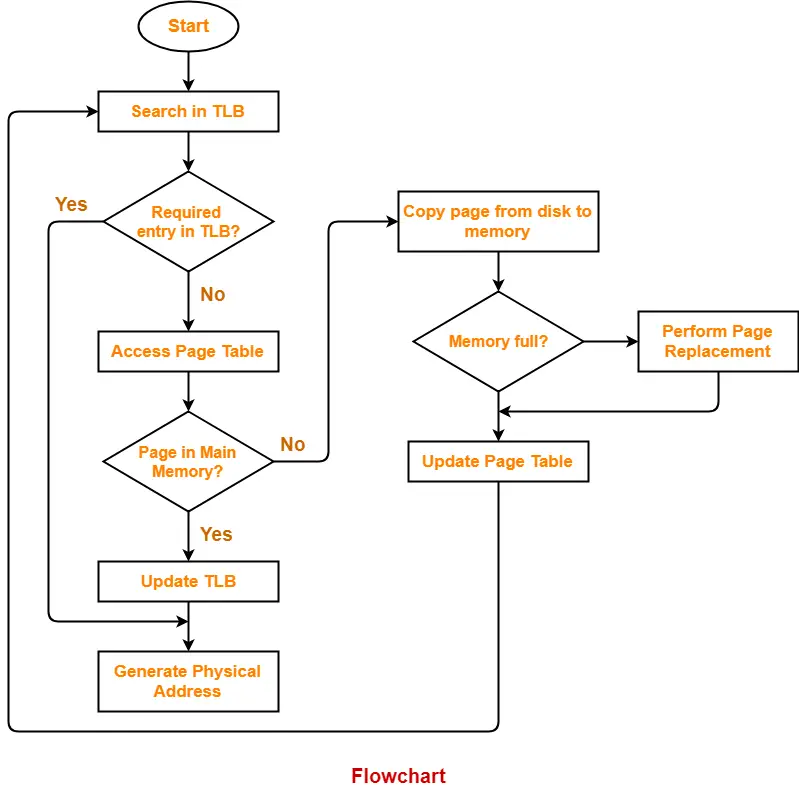## Page Fault Service Time-

• The time taken by the page fault handling routine to service the page fault is called as page fault service time.
• Page fault service time is much greater than main memory access time.
• It increases the effective access time.

To gain better understanding about Page Fault in OS,

Watch this Video Lecture

Next Article- Page Replacement Algorithms

Get more notes and other study material of Operating System.

Watch video lectures by visiting our YouTube channel LearnVidFun.